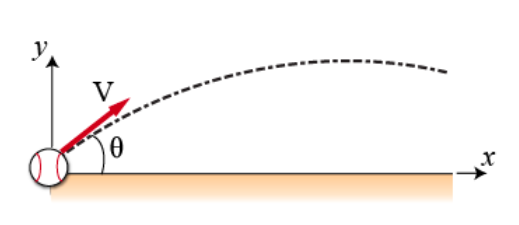# Problem: During a baseball game, a baseball is struck at ground level by a batter. The ball leaves the baseball bat with an initial velocity v0 = 25 m/s at an angle θ = 15° above horizontal. Let the origin of the Cartesian coordinate system be the ball's position the instant it leaves the bat. Air resistance may be ignored throughout this problem.(a) Express the magnitude of the ball's initial horizontal velocity v0x in terms of v0 and θ.(b) Express the magnitude of the ball's initial vertical velocity v0y in terms of v0 and θ.(c) Find the ball's maximum vertical height hmax in meters above the ground.

###### FREE Expert Solution

2D vectors Components:

The maximum height reached:

$\overline{){{\mathbf{h}}}_{\mathbf{m}\mathbf{a}\mathbf{x}}{\mathbf{=}}\frac{{{\mathbf{v}}_{\mathbf{0}\mathbit{y}}}^{\mathbf{2}}}{\mathbf{2}\mathbf{g}}}$

(a)

From the vectors components equation:

v0x = v0cosθ

The magnitude of the ball's initial horizontal velocity is v0cosθ.

82% (311 ratings)###### Problem Details

During a baseball game, a baseball is struck at ground level by a batter. The ball leaves the baseball bat with an initial velocity v0 = 25 m/s at an angle θ = 15° above horizontal. Let the origin of the Cartesian coordinate system be the ball's position the instant it leaves the bat. Air resistance may be ignored throughout this problem.

(a) Express the magnitude of the ball's initial horizontal velocity v0x in terms of v0 and θ.
(b) Express the magnitude of the ball's initial vertical velocity v0y in terms of v0 and θ.
(c) Find the ball's maximum vertical height hmax in meters above the ground.